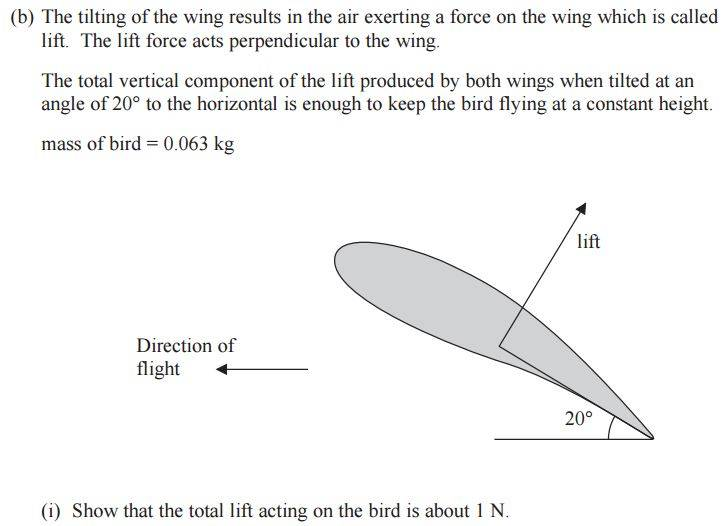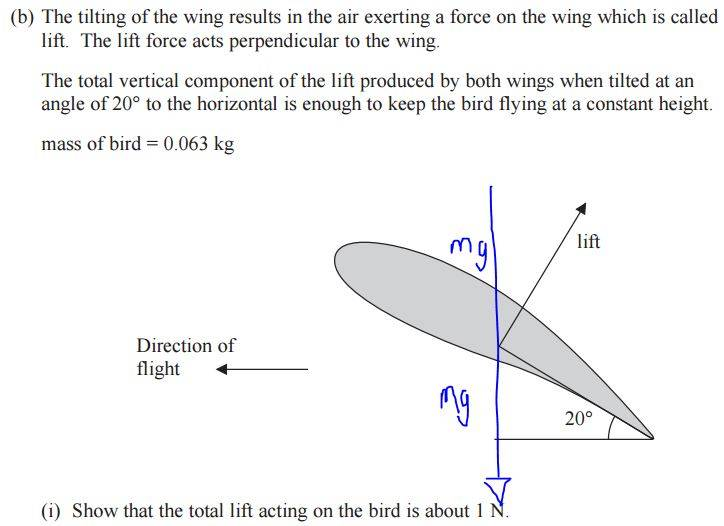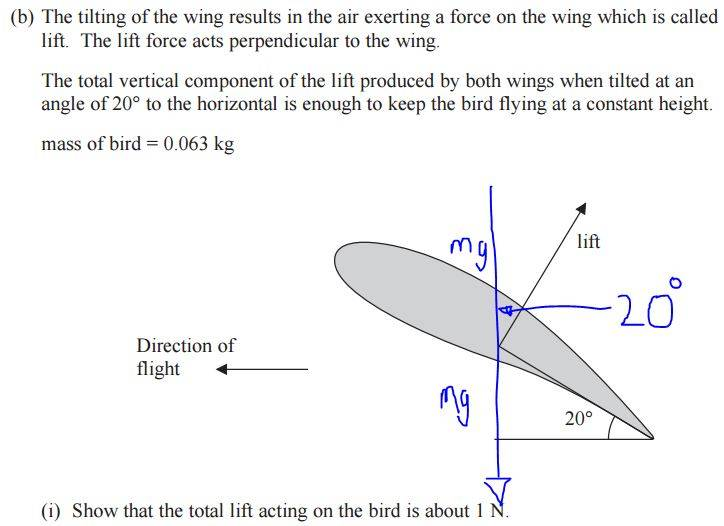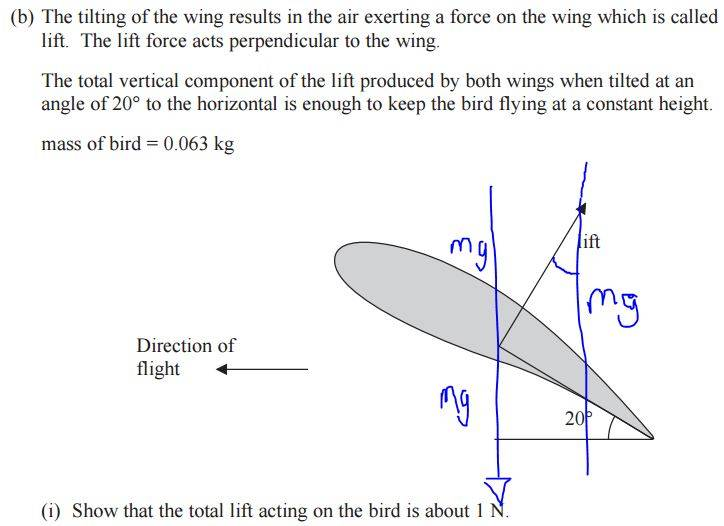# Perpendicular forces## The Attempt at a Solution

[/B]
I was of the understanding that the slope can be calculated as mgsin20 whereas the force acting straight down through the lift is mgcos20 but the answer is mg divided by cos 20??

Doc Al
Mentor
Hint: What force components act in the vertical direction? What must they add to?

•ravsterphysics
Hint: What force components act in the vertical direction? What must they add to?

in the vertical direction we have weight acting downwards and lift acting upwards? so does it look like this?Doc Al
Mentor
in the vertical direction we have weight acting downwards and lift acting upwards?
The weight acts downward. Good! But only a component of the lift force acts vertically. What is that component?

•ravsterphysics
The weight acts downward. Good! But only a component of the lift force acts vertically. What is that component?

So only the 'top' part of the weight is what we're after? And the angle is also 20 degrees? So it looks like this?in which case lift would then be Lift = mg/cos20

I see!

In that case, how does this differ to my notes that say the perpendicular force to the slope would be mgcos20? Is it because we're dealing with a component only?

Doc Al
Mentor
So only the 'top' part of the weight is what we're after?
Not sure what you mean. There are two forces acting on the wing: Weight, which acts down. And the lift force, which acts at the angle shown.

You need to analyze the vertical components.

And the angle is also 20 degrees?
The angle that the lift force makes with the vertical is 20 degrees. So what is the vertical component of that force?

•ravsterphysics
Not sure what you mean. There are two forces acting on the wing: Weight, which acts down. And the lift force, which acts at the angle shown.

You need to analyze the vertical components.

The angle that the lift force makes with the vertical is 20 degrees. So what is the vertical component of that force?

The vertical component is weight (mg) right? So to get lift, we use trig to end up with Lift = mg/cos20? Is that what you mean by vertical component?Doc Al
Mentor
The vertical component is weight (mg) right? So to get lift, we use trig to end up with Lift = mg/cos20? Is that what you mean by vertical component?
No. I simply mean: What is the component of the lift force in the vertical direction? You know the angle to the vertical, so how would you find the vertical component? (It will be in terms of L. You'll then use it to solve for L.)

•ravsterphysics
No. I simply mean: What is the component of the lift force in the vertical direction? You know the angle to the vertical, so how would you find the vertical component? (It will be in terms of L. You'll then use it to solve for L.)

okay now i'm lost, i thought the mg/cos20 IS the vertical component? if not, can you write it, it'll probably click for me that way.

Doc Al
Mentor

i thought the mg/cos20 IS the vertical component?
Not quite.

in which case lift would then be Lift = mg/cos20
You had this correct! (Didn't see it earlier.)

But to be clear, here's how to figure out what L is.
(1) vertical component of L = L cos20 (This is what I was trying to get you to say!)
(2) vertical component of weight is just mg (of course, since it's vertical)

ΣFy = 0
L cos20 - mg = 0

Thus:
L cos20 = mg
L = mg/cos20

Does that make sense?

•ravsterphysics
haruspex
•